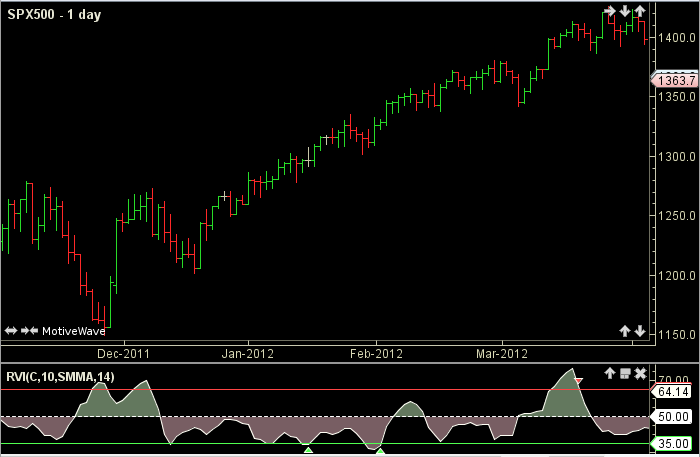# Using RVI to filter/entry tops and bottoms - page 2160157

tampa:
Do we have this for mt (Relative Volatility Index) :Formula here : Relative Volatility Index

There is Dorsey's inertia (here : https://www.mql5.com/en/forum/179802 ) but I did not find "Relative Volatility Index" yet2047

There is Dorsey's inertia (here : https://www.mql5.com/en/forum/179802 ) but I did not find "Relative Volatility Index" yet

Thanks

Can Relative Volatility Index be made?160157

tampa:
Thanks Can Relative Volatility Index be made?

Will check what can be done160157

tampa:
Thanks Can Relative Volatility Index be made?

that is actually an rsi of standard deviation - will be made tomorrow2047

that is actually an rsi of standard deviation - will be made tomorrow

OK. Thanks ....160157

tampa:
OK. Thanks ....

Here is relative volatility index made according to that formula found there : relative_volatility_index.mq4

There is one deviation : I did not know if that coding language inherits the previous state of the variables or not (some coding languages, like easy language do that, some don't) so one parameter is added : InheritPrevious. In the example upper is when InheritPrevious is set to false and lower when it is set to true

Files:
rvi.gif 66 kb2047

Here is relative volatility index made according to that formula found there : relative_volatility_index.mq4 There is one deviation : I did not know if that coding language inherits the previous state of the variables or not (some coding languages, like easy language do that, some don't) so one parameter is added : InheritPrevious. In the example upper is when InheritPrevious is set to false and lower when it is set to true

ThanksWill test it2047